# Editing Raster Data

## Producer Field Guide

HGD_Product
Producer Field Guide
HGD_Portfolio_Suite
Producer

ERDAS IMAGINE provides raster editing tools for editing the data values of thematic and continuous raster data. This is primarily a correction mechanism for you to correct bad data values which produce noise, such as spikes and holes in imagery. The raster editing functions can be applied to the entire image or a user-selected area of interest (AOI).

With raster editing, data values in thematic data can also be recoded according to class. Recoding is a function that reassigns data values to a region or to an entire class of pixels.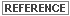See Geographic Information Systems for information about recoding data. See Enhancement for information about reducing data noise using spatial filtering.

The ERDAS IMAGINE raster editing functions allow the use of focal and global spatial modeling functions for computing the values to replace noisy pixels or areas in continuous or thematic data.

Focal operations are filters that calculate the replacement value based on a window (3 × 3, 5 × 5, and so forth), and replace the pixel of interest with the replacement value. Therefore this function affects one pixel at a time, and the number of surrounding pixels that influence the value is determined by the size of the moving window.

Global operations calculate the replacement value for an entire area rather than affecting one pixel at a time. These functions, specifically the Majority option, are more applicable to thematic data.See ERDAS IMAGINE Help for using and selecting AOIs.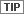Raster editing tools are available in the IMAGINE Workspace.

Editing Continuous (Athematic) Data

Editing DEMs

DEMs occasionally contain spurious pixels or bad data. These spikes, holes, and other noises caused by automatic DEM extraction can be corrected by editing the raster data values and replacing them with meaningful values. This discussion of raster editing focuses on DEM editing.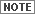ERDAS IMAGINE Raster Editing functionality was originally designed to edit DEMs, but it can also be used with images of other continuous data sources, such as radar, SPOT, Landsat, and digitized photographs.

When editing continuous raster data, you can modify or replace original pixel values with the following:

• a constant value—enter a known constant value for areas such as lakes.
• average of the buffering pixels—replace the original pixel value with the average of the pixels in a specified buffer area around the AOI. This is used where the constant values of the AOI are not known, but the area is flat or homogeneous with little variation (for example, a lake).
• original data value plus a constant value—add a negative constant value to the original data values to compensate for the height of trees and other vertical features in the DEM. This technique is commonly used in forested areas.
• spatial filtering—filter data values to eliminate noise such as spikes or holes in the data.
• interpolation techniques.

Interpolation Techniques

While the previously listed raster editing techniques are perfectly suitable for some applications, the following interpolation techniques provide the best methods for raster editing:

• 2-D polynomial—surface approximation
• multisurface functions—with least squares prediction
• distance weighting

Each pixel’s data value is interpolated from the reference points in the data file. These interpolation techniques are described below:

2-D Polynomial

This interpolation technique provides faster interpolation calculations than distance weighting and multisurface functions. The following equation is used: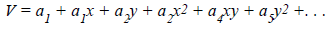Where:

V = data value (elevation value for DEM)

a = polynomial coefficients

x = x coordinate

y = y coordinate

Multisurface Functions

The multisurface technique provides the most accurate results for editing DEMs that have been created through automatic extraction. The following equation is used: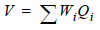Where:

V = output data value (elevation value for DEM)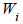= coefficients which are derived by the least squares method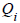= distance-related kernels which are actually interpretable as continuous single value surfaces

Source: Wang, Z., 1990

Distance Weighting

The weighting function determines how the output data values are interpolated from a set of reference data points. For each pixel, the values of all reference points are weighted by a value corresponding with the distance between each point and the pixel.

The weighting function used in ERDAS IMAGINE is: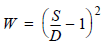Where:

S = normalization factor

D = distance from output data point and reference point

The value for any given pixel is calculated by taking the sum of weighting factors for all reference points multiplied by the data values of those points, and dividing by the sum of the weighting factors: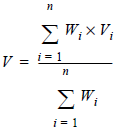Where:

V = output data value (elevation value for DEM)

i =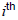reference point= weighting factor of point i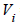= data value of point i

n = number of reference points

Source: Wang, Z., 1990